# 毕业设计必备案例：Python开发桌面程序——各种版本学生信息管理系统

Python48``````students = [
{'name': '张三', 'chinese': 60, 'math': 60, 'english': 60},
{'name': '李四', 'chinese': 60, 'math': 60, 'english': 60},
{'name': '王五', 'chinese': 60, 'math': 60, 'english': 60},
]

while True:
print(msg)
action = input('请选择你想要进行的操作：')
# action = 1 2 3 4 5 0
print('选择的操作:\t', action)

if action == 1:
print('1. 新建学生信息')
# 新建学员信息
name = input('请输入姓名:')
chinese = int(input('请输入语文成绩:'))
math = int(input('请输入数学成绩:'))
english = int(input('请输入英语成绩:'))
# 总分要输入吗 ?
new_student = {'name': name, 'chinese': chinese, 'math': math, 'english': english}
# 新的学员信息有了,怎么加到程序里面去
students.append(new_student)
elif action == 2:
print('2. 显示全部信息')
# 拿到所有学员的信息
print('姓名        语文        数学        英语        总分')
for student in students:
# print(student)
print(student['name'] + '        ' +
str(student['chinese']) + '        ' +
str(student['math']) + '        ' +
str(student['english']) + '        ' +
str(student['chinese'] + student['math'] + student['english']))
elif action == 3:
print('3. 查询学生信息')
name = input('请输入需要查询的学员姓名:')

# 然后打印找到的学员,没有找到就打印此学员不存在

flag = False  # 定义一个变量记录是否找到了学员
# 1. 遍历所有的学员
for student in students:
# print(student)
# 2. 如果输入的学员姓名与代码中的学员姓名一样,就是找到了
if student['name'] == name:
# 3. 然后打印找到的学员
print('姓名        语文        数学        英语        总分')
print(student['name'] + '        ' +
student['chinese'] + '        ' +
student['math'] + '        ' +
student['english'] + '        ' +
student['chinese'] + student['math'] + student['english'])
flag = True  # 找到了之后就标记一下
# 如果没有找到就打印此学员不存在
if not flag:
# 4. 没有找到就打印此学员不存在
# 应该是找完所有学员都没有找到之后才打印
print('此学员不存在')
# 一个程序员的能力与他敲过的代码是成正比的

elif action == 4:
print('4. 删除学生信息')
# 输入需要删除的学生姓名
name = input('请输入需要删除的学员姓名:')
# 找到需要删除的学员
flag = False
for student in students:
if student['name'] == name:
# 找到之后直接删除学员
# 如何从列表中删除元素
students.remove(student)
print(student['name'] + ' 学员删除成功')
flag = True
if not flag:
print('此学员不存在，无法删除')

elif action == 5:
print('5. 修改学生信息')
name = input('请输入需要修改的学员姓名:')
# 找到需要删除的学员
flag = False
for student in students:
if student['name'] == name:
# 找到学员之后就可以进行修改
print('(输入为空则不修改)')
# input 输入可能会为空
name = input('请重新输入姓名:')
chinese = input('请重新输入语文成绩:')
math = input('请重新输入数学成绩:')
english = input('请重新输入英语成绩:')
# 如果  name 输入了内容,我们再进行复制
if name:  # true false
student['name'] = name
# 需要把字符串转化为数字
if chinese:
student['chinese'] = int(chinese)
if math:
student['math'] = int(math)
if english:
student['english'] = int(english)

# 建立知识体系 需要一点一点的进行积累
flag = True
if not flag:
print('此学员不存在，无法修改')

elif action == 6:
# 条件查询 总分  语文+数据
# > < =
print()
elif action == '0':
print('0. 退出系统 欢迎下次光临')
break
else:
print('请选择正确的操作')
``````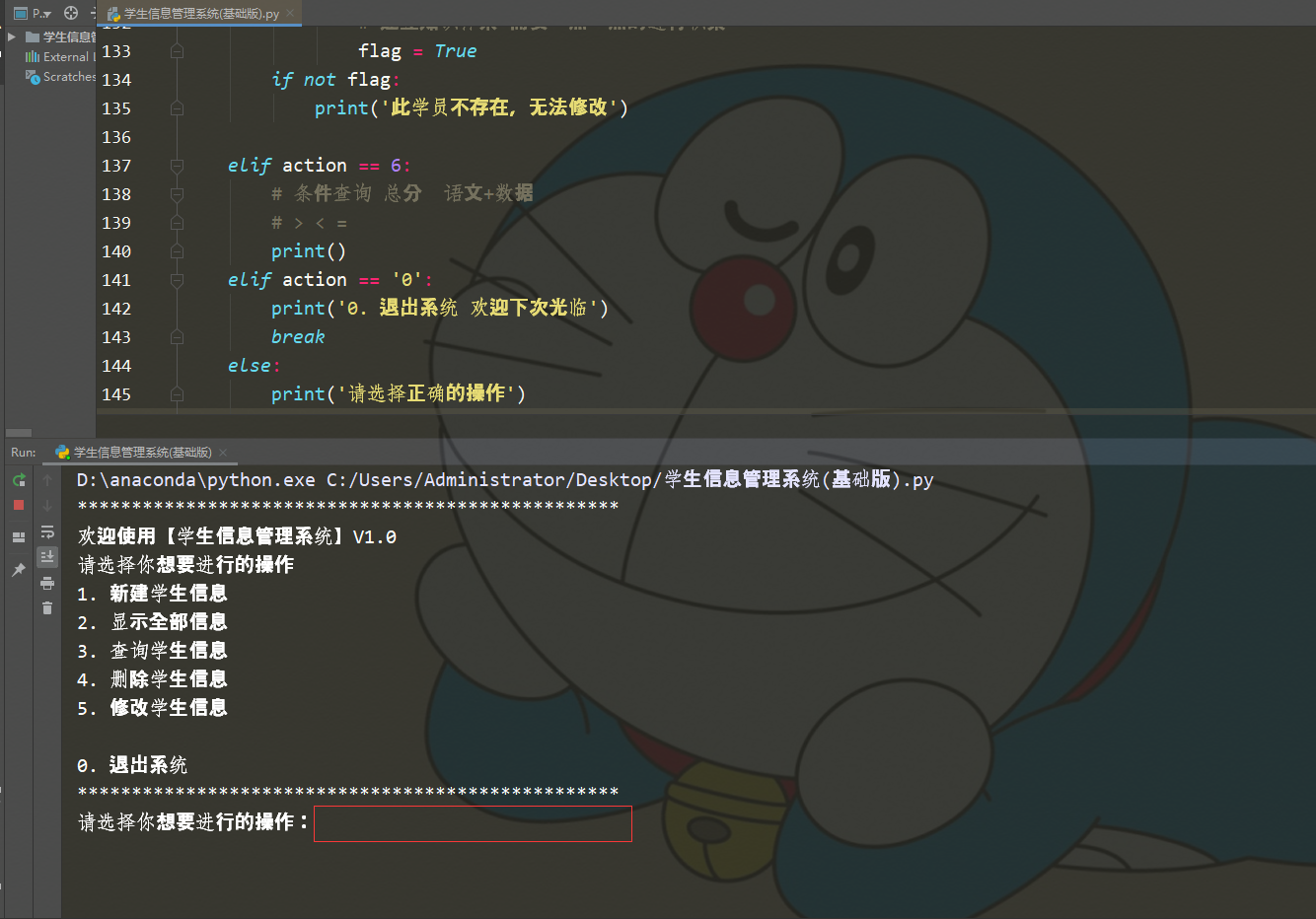``````#兄弟们学习python，有时候不知道怎么学，从哪里开始学。掌握了基本的一些语法或者做了两个案例后，不知道下一步怎么走，不知道如何去学习更加高深的知识。
#那么对于这些大兄弟们，我准备了大量的免费视频教程，PDF电子书籍，以及视频源的源代码！
#还会有大佬解答！
#都在这个裙里了 872937351
#欢迎加入，一起讨论 一起学习！
``````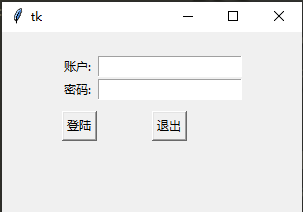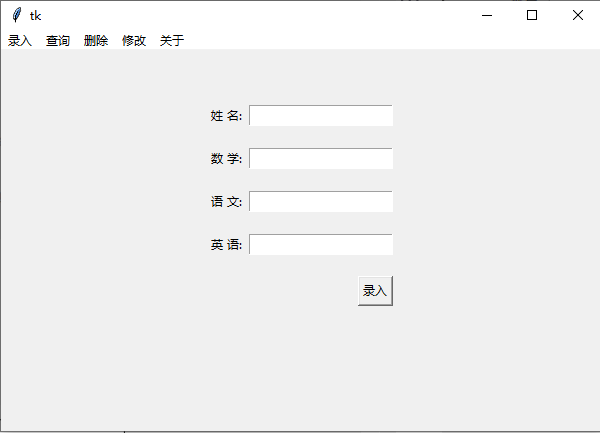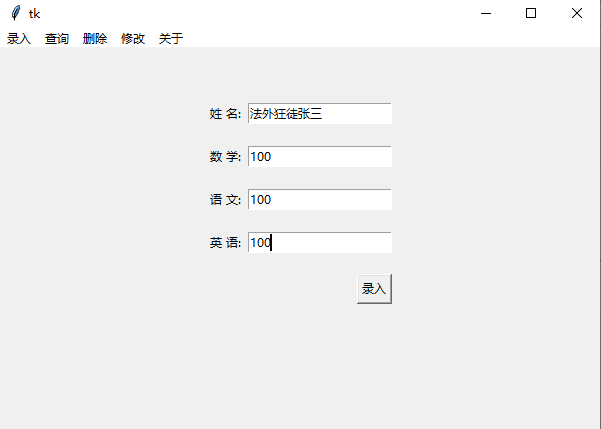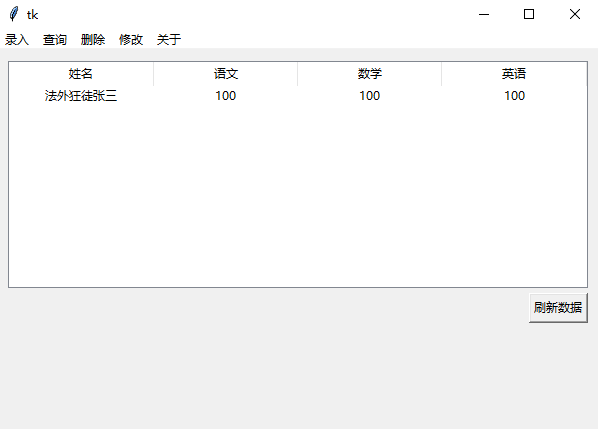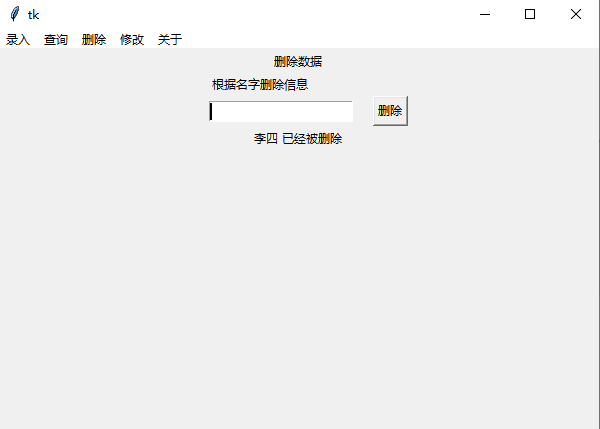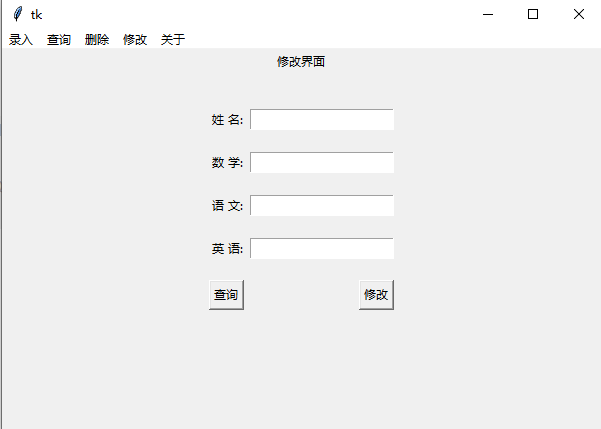Original: https://www.cnblogs.com/hahaa/p/15499177.html
Author: 轻松学Python
Title: 毕业设计必备案例：Python开发桌面程序——各种版本学生信息管理系统